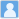• ### Theory of remaining energy —wave mechanics of particles in curved space-time(Part III)

分类： 物理学 >> 地球物理学、天文学和天体物理学 提交时间： 2023-03-25Yu Xianqiao

摘要：In this article, based on the wave equation established in the first part of this series, I have studied the evolution of the energy density of the universe from the initial moment to the present, and found that there is still residual energy of the initial energy of the universe, which appears in the form of vacuum energy. The residual energy is the simplest explanation for dark matter. The calculations show that the present average energy of each momentum mode in the residual energy is of the order of 10^{-3} eV, which is approximately 3 to 5 times the averaged energy of photons in the cosmic microwave background.

• ### The source of cosmological constant —wave mechanics of particles in curved space-time(Part II)

分类： 物理学 >> 地球物理学、天文学和天体物理学 提交时间： 2023-03-13Yu Xianqiao

摘要：In this article, we will see that the existence of cosmological constant is a natural possible result of the new wave equation, which has been established in the first part of this series. After analyzing various possible sources of cosmological constant, the most likely conclusion is drawn that the cosmological constant is not from the vacuum energy of matter, but from space itself, it is an inherent essential feature of space itself.

• ### Wave equation—wave mechanics of particles in curved space-time(Part I)

提交时间： 2023-03-13Yu Xianqiao

摘要： In this paper, a universal wave equation of the motion of microscopic particles in curved space-time is established, which satisfies the conservation of probability. Under the classical limit conditions, the wave equation returns to the dynamic equation of special relativity. The application of the wave equation in the evolution of a flat expanding universe has preliminarily proved the correctness of the equation, and the new wave equation may open a window for us to understand physics within the Planck scale.